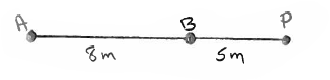# Problem: Two antennas A and B radiate electromagnetic waves that are in phase and have frequency f. Antenna A is 8.00 m to the left of antenna  B and point P is 5.00 m to the right of antenna B. What is the smallest value of the frequency  f for which the waves from the two antennas have destructive interference at point P?

###### FREE Expert Solution
97% (254 ratings)
###### Problem Details

Two antennas A and B radiate electromagnetic waves that are in phase and have frequency f. Antenna A is 8.00 m to the left of antenna  B and point P is 5.00 m to the right of antenna B. What is the smallest value of the frequency  f for which the waves from the two antennas have destructive interference at point P?